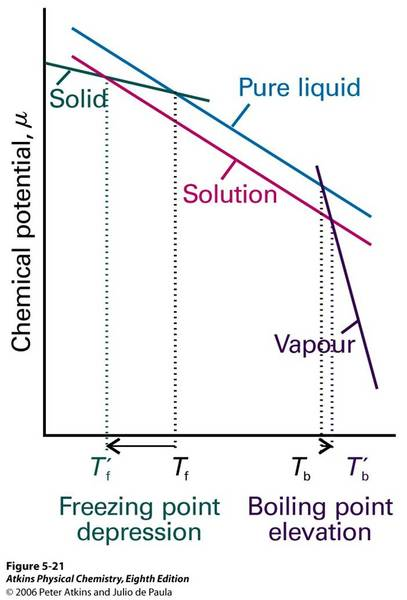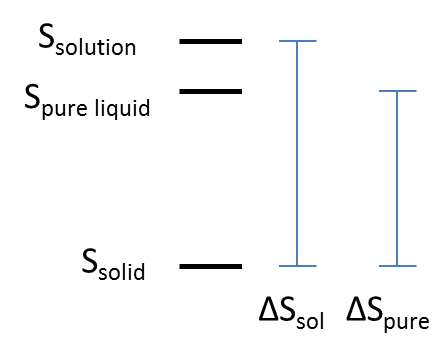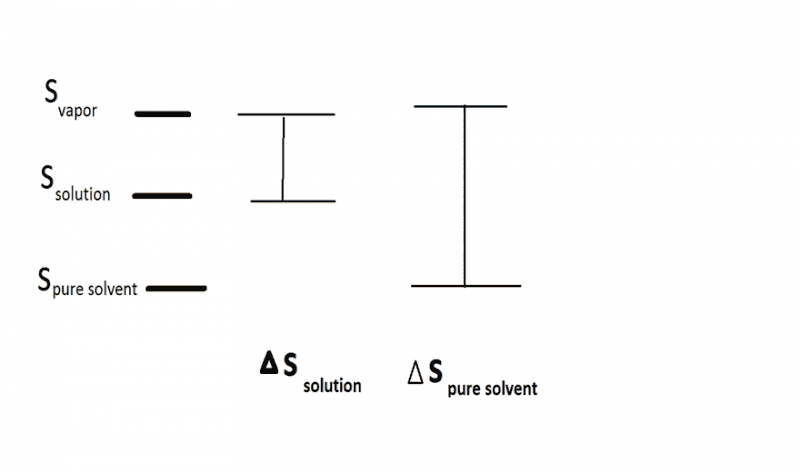# Freezing point depression

siddharth23
I got only a part of it. Did you understand the colligative property of freezing point depression?

Homework Helper
Gold Member
In this thread https://www.physicsforums.com/threads/freezing-point-depression.275386/ in ygggdrasil answer I didn't understand this line This greater loss of entropy leads to a lower freezing point.

If you look in a good quality Physical Chemistry textbook you may find a picture that helps. Look for a figure showing the chemical potential of solvent as a function of temperature.

At higher temperature, you will see a line with a large negative slope. This is the line for the chemical potential of vapor. At lower temperatures, you will see a line with a slightly less negative slope (the liquid line) , and at even lower temperatures you will see a line with an even less negative slope (the solid line). The places where the lines intersect show the transition temperatures (boiling and freezing). Adding solute to the solvent lowers the chemical potential of the liquid. The vapour and solid lines remain unchanged. This sliding down of the solution line (compared to that for the pure liquid) is the result of the increased entropy (decreased chemical potential) in the solution compared to isolated pure solvent and isolated pure solute.

As a result of the lowering of the liquid line, you see the intersection between solid and liquid curves being pushed to lower temperature (the freezing point is depressed), while the intersection of the liquid-vapour lines is pushed to higher temperature -- the boiling point is elevated.

Here is a figure from the web:Last edited:
•Greg Bernhardt
Gold Member
2021 Award
Quantum Defect's answer covers your question nicely, but here is an alternate explanation that tracks more closely with my explanation in the previous post.

As I said in my previous post, the freezing point is set by a competition between the lower potential energy associated with the solid state and the higher entropy associated with the liquid state. The free energy change of freezing is given by ΔG = ΔH - TΔS. At the freezing point Tf, the solid and liquid phases are at equilibrium, so ΔG = 0 = ΔH - TfΔS. Rearranging this equation gives Tf = ΔH/ΔS. Thus, if ΔS increases, the freezing point should decrease.

How do the ΔH and ΔS of freezing change when freezing a pure liquid versus a solution? ΔH is roughly the same for both processes, assuming an ideal solution. However, the overall entropy of a solution is higher than the overall entropy of a pure liquid. This makes the overall ΔS associated with freezing larger when freezing a solution than when freezing a pure liquid (see the figure below).•gracy
gracy
This was extremely helpful.I want one more favor of yours regarding boiling point elevation.These lines of your previous post
" Adding a solute to the solvent raises the entropy of the solution, which decreases the gain in entropy from going from liquid to gas. Because the gain in entropy is lesser, the competition between entropy and enthalapy is shifted more in favor of the liquid so that the boiling point increases and hence the vapor pressure decreases"

Gold Member
2021 Award
This was extremely helpful.I want one more favor of yours regarding boiling point elevation.These lines of your previous post
" Adding a solute to the solvent raises the entropy of the solution, which decreases the gain in entropy from going from liquid to gas. Because the gain in entropy is lesser, the competition between entropy and enthalapy is shifted more in favor of the liquid so that the boiling point increases and hence the vapor pressure decreases"

The reasoning is similar to the case for freezing point depression. Try drawing out a similar diagram with the entropies of the pure liquid, solution, and vapor, then figure out which is greater, ΔS for boiling the pure liquid or ΔS for boiling the solution.

gracy
Yes,you are right.I figured it out.. The free energy change of boiling is given by ΔG = ΔH - TΔS. At the boiling point Tb, the liquid and vapor phases are at equilibrium, so ΔG = 0 = ΔH - TbΔS. Rearranging this equation gives Tb = ΔH/ΔS. Thus, if ΔS decreases the boiling point should increase.
Solute is added to pure solvent to form a solution ,entropy of solution is greater than entropy of pure solvent.Entropy of vapor phase is greater than both solvent as well as solution.As ΔS for solution to vapor is smaller than ΔS for pure solvent to vapor,therefor Tb(boiling point )of solution is greater than boiling point of pure solvent because of this equation
Tb = ΔH/ΔS. Thus, if ΔS decreases the boiling point should increase.This increase of boiling point on adding solute particles is called "boiling point elevation".
Right?

•Ygggdrasil